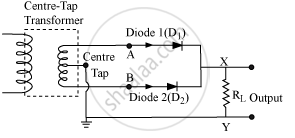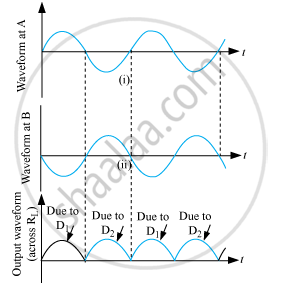# Answer the Following Question. Draw the Circuit Diagram of a Full-wave Rectifier and Explain Its Working. Also, Give the Input and Output Waveforms. - Physics

Draw the circuit diagram of a full-wave rectifier and explain its working. Also, give the input and output waveforms.

#### Solution

Full-wave rectifier
Two diodes are used to give rectified O/P corresponding to both positive as well as negative half cycles.When the voltage at A with respect to the center tap is positive, and the voltage at B is negative. Then, D1 is forward biased and D2 is reversed biased. Hence, D1 conducts and D2 does not. When the voltage of A becomes negative, then B becomes +ve. Therefore, D1 does not conduct and D2 conducts. Hence, we obtain output voltage during both the positive as well as negative half of the cycle.
Input and Output waveforms are shown below.Concept: Diode as a Rectifier
Is there an error in this question or solution?